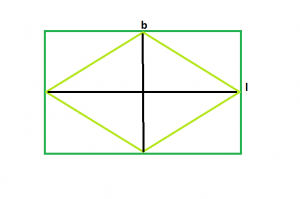Related Articles

# Area of the biggest possible rhombus that can be inscribed in a rectangle

• Last Updated : 15 Mar, 2021

Given a rectangle of length l and breadth b, the task is to find the largest rhombus that can be inscribed in the rectangle.
Examples

```Input : l = 5, b = 4
Output : 10

Input : l = 16, b = 6
Output : 48```From the figure, we can see, the biggest rhombus that could be inscribed within the rectangle will have its diagonals equal to the length & breadth of the rectangle.
So, Area of rhombus, A = (l*b)/2
Below is the implementation of the above approach:

## C++

 `// C++ Program to find the biggest rhombus``// which can be inscribed within the rectangle``#include ``using` `namespace` `std;` `// Function to find the area``// of the biggest rhombus``float` `rhombusarea(``float` `l, ``float` `b)``{``    ``// the length and breadth cannot be negative``    ``if` `(l < 0 || b < 0)``        ``return` `-1;` `    ``// area of the rhombus``    ``return` `(l * b) / 2;``}` `// Driver code``int` `main()``{``    ``float` `l = 16, b = 6;``    ``cout << rhombusarea(l, b) << endl;``    ``return` `0;``}`

## Java

 `// Java Program to find the``// biggest rhombus which can be``// inscribed within the rectangle``import` `java.io.*;` `class` `GFG``{` `// Function to find the area``// of the biggest rhombus``static` `float` `rhombusarea(``float` `l,``                         ``float` `b)``{``    ``// the length and breadth``    ``// cannot be negative``    ``if` `(l < ``0` `|| b < ``0``)``        ``return` `-``1``;` `    ``// area of the rhombus``    ``return` `(l * b) / ``2``;``}` `// Driver code``public` `static` `void` `main (String[] args)``{``    ``float` `l = ``16``, b = ``6``;``    ``System.out.println(rhombusarea(l, b));``}``}` `// This code is contributed``// by inder_verma`

## Python3

 `# Python 3 Program to find the biggest rhombus``# which can be inscribed within the rectangle`  `# Function to find the area``# of the biggest rhombus``def` `rhombusarea(l,b):``    ``# the length and breadth cannot be negative``    ``if` `(l < ``0` `or` `b < ``0``):``        ``return` `-``1` `    ``# area of the rhombus``    ``return` `(l ``*` `b) ``/` `2` `# Driver code``if` `__name__ ``=``=` `'__main__'``:``    ``l ``=` `16``    ``b ``=` `6``    ``print``(rhombusarea(l, b))`

## C#

 `// C# Program to find the``// biggest rhombus which can be``// inscribed within the rectangle``using` `System;` `class` `GFG``{` `// Function to find the area``// of the biggest rhombus``static` `float` `rhombusarea(``float` `l,``                        ``float` `b)``{``    ``// the length and breadth``    ``// cannot be negative``    ``if` `(l < 0 || b < 0)``        ``return` `-1;` `    ``// area of the rhombus``    ``return` `(l * b) / 2;``}` `// Driver code``public` `static` `void` `Main ()``{``    ``float` `l = 16, b = 6;``    ``Console.WriteLine(rhombusarea(l, b));``}``}` `// This code is contributed``// by shs`

## PHP

 `

## Javascript

 ``
Output:
`48`

Attention reader! Don’t stop learning now. Join the First-Step-to-DSA Course for Class 9 to 12 students , specifically designed to introduce data structures and algorithms to the class 9 to 12 students

My Personal Notes arrow_drop_up